# 240 373 Image Processing Montri Karnjanadecha montricoe psu

• Slides: 19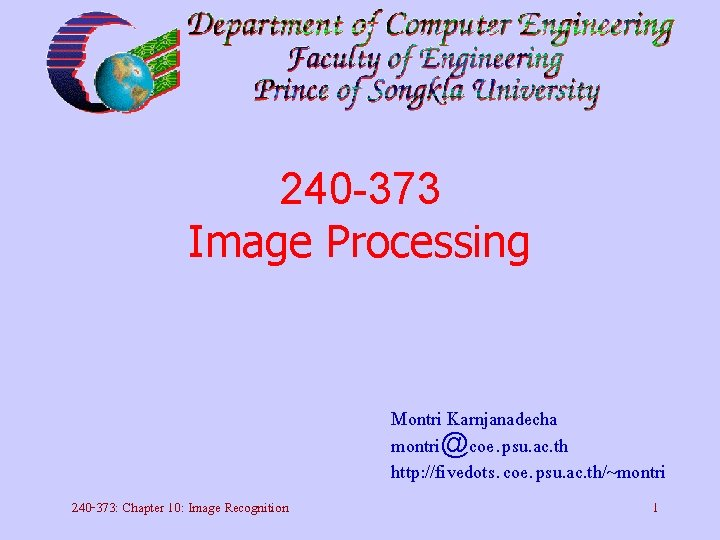240 -373 Image Processing Montri Karnjanadecha [email protected] psu. ac. th http: //fivedots. coe. psu. ac. th/~montri 240 -373: Chapter 10: Image Recognition 1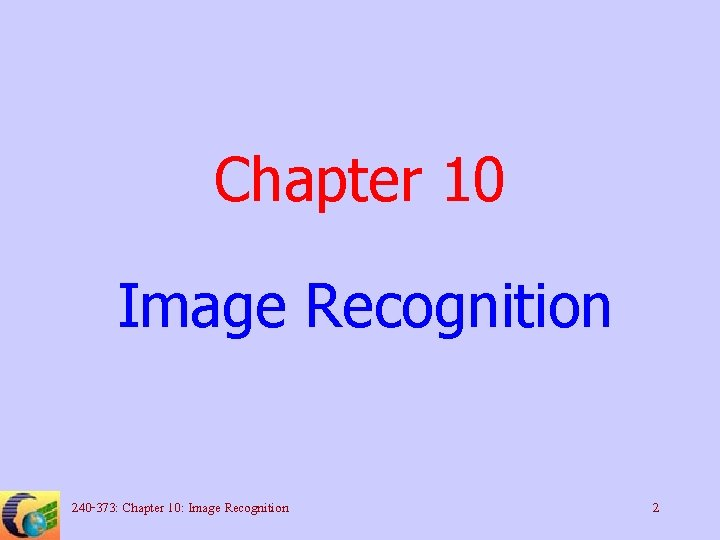Chapter 10 Image Recognition 240 -373: Chapter 10: Image Recognition 2Pattern Recognition and Training • Pattern -- set of values (known size) that describe things • The general problem 240 -373: Chapter 10: Image Recognition 3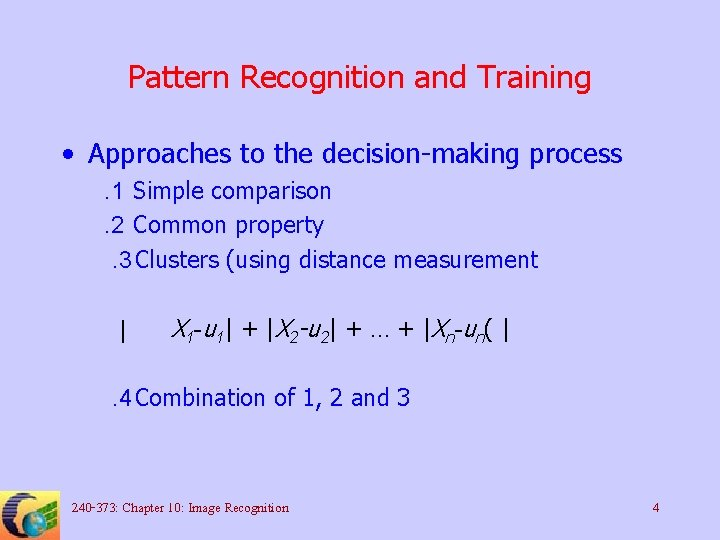Pattern Recognition and Training • Approaches to the decision-making process. 1 Simple comparison. 2 Common property. 3 Clusters (using distance measurement | X 1 -u 1| + |X 2 -u 2| + … + |Xn-un( | . 4 Combination of 1, 2 and 3 240 -373: Chapter 10: Image Recognition 4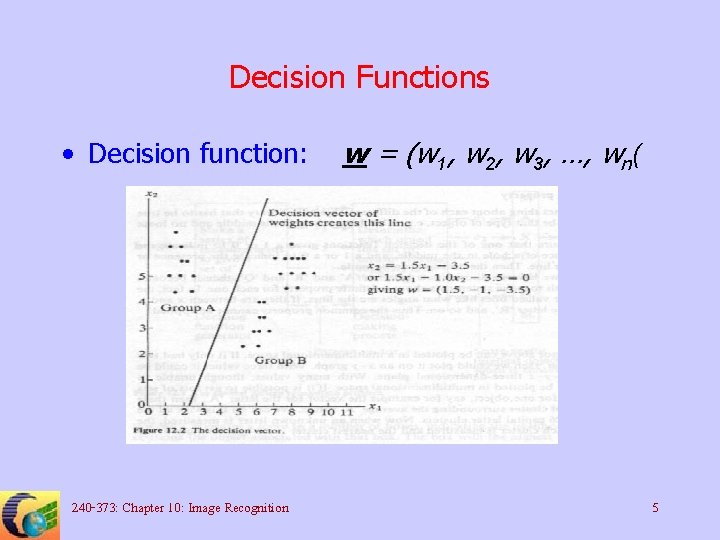Decision Functions • Decision function: 240 -373: Chapter 10: Image Recognition w = (w 1, w 2, w 3, …, wn( 5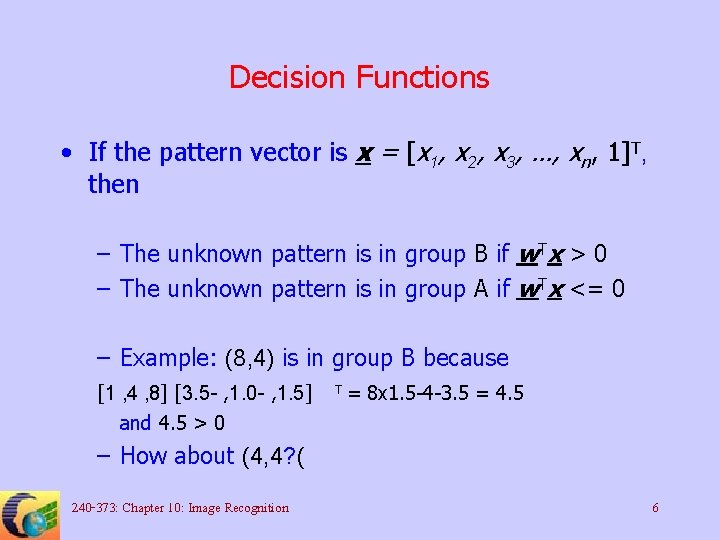Decision Functions • If the pattern vector is x = [x 1, x 2, x 3, …, xn, 1]T, then – The unknown pattern is in group B if w. Tx > 0 – The unknown pattern is in group A if w. Tx <= 0 – Example: (8, 4) is in group B because [1 , 4 , 8] [3. 5 - , 1. 0 - , 1. 5] and 4. 5 > 0 T = 8 x 1. 5 -4 -3. 5 = 4. 5 – How about (4, 4? ( 240 -373: Chapter 10: Image Recognition 6Decision Functions (Cont’d( • The number of groups can be more than 2 240 -373: Chapter 10: Image Recognition 7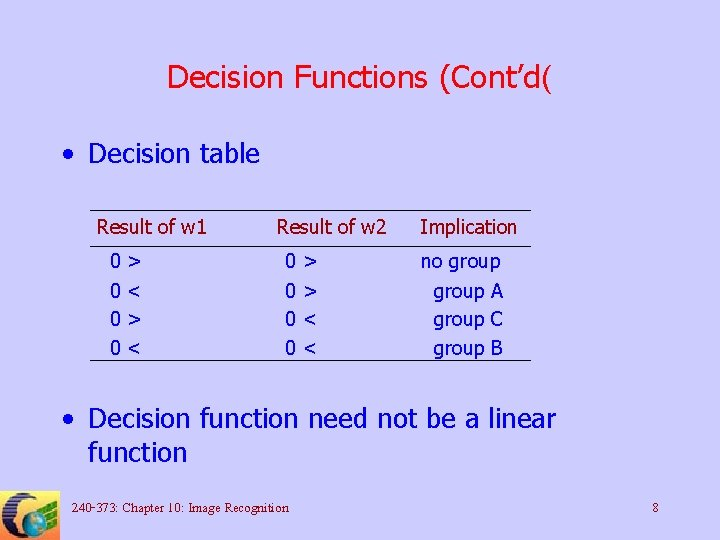Decision Functions (Cont’d( • Decision table Result of w 1 0 0 > < Result of w 2 0 0 > > < < Implication no group A group C group B • Decision function need not be a linear function 240 -373: Chapter 10: Image Recognition 8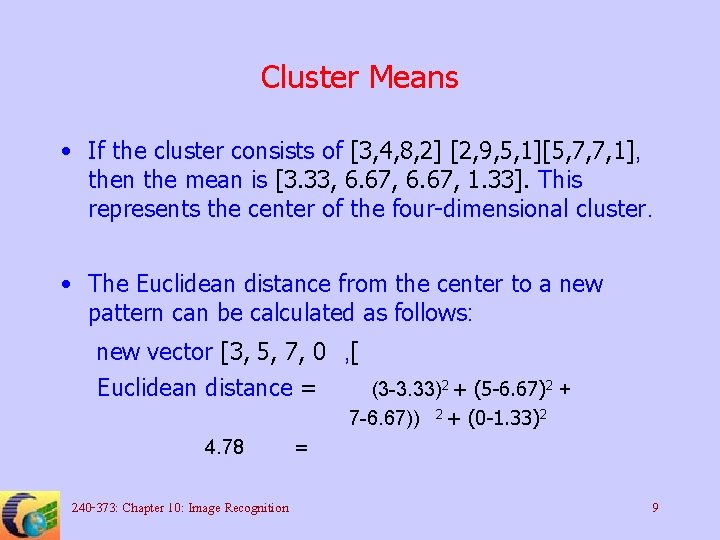Cluster Means • If the cluster consists of [3, 4, 8, 2] [2, 9, 5, 1][5, 7, 7, 1], then the mean is [3. 33, 6. 67, 1. 33]. This represents the center of the four-dimensional cluster. • The Euclidean distance from the center to a new pattern can be calculated as follows: new vector [3, 5, 7, 0 , [ Euclidean distance = (3 -3. 33)2 + (5 -6. 67)2 + 7 -6. 67)) 4. 78 240 -373: Chapter 10: Image Recognition 2+ (0 -1. 33)2 = 9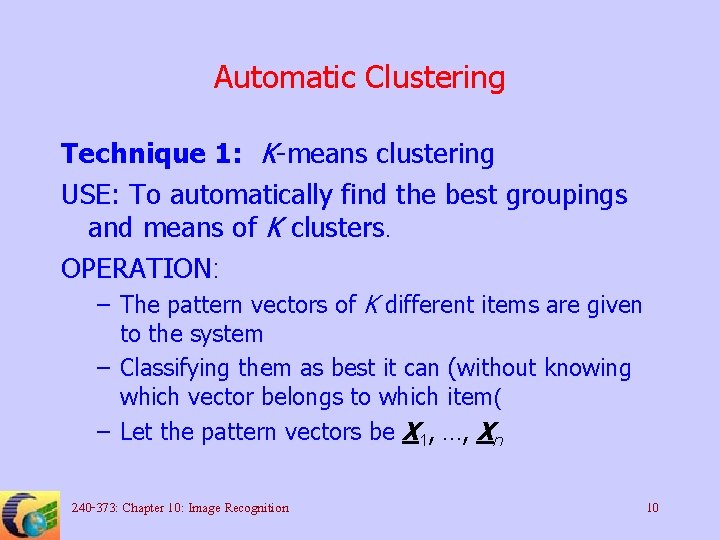Automatic Clustering Technique 1: K-means clustering USE: To automatically find the best groupings and means of K clusters. OPERATION: – The pattern vectors of K different items are given to the system – Classifying them as best it can (without knowing which vector belongs to which item( – Let the pattern vectors be X 1, …, Xn 240 -373: Chapter 10: Image Recognition 10Automatic Clustering OPERATION: (cont’d( – Take the first K points as the initial estimation of the cluster means M 1 = X 1, M 2 = X 2, …, Mk = Xk * Allocate each pattern vector to the nearest group (minimum distance( – Calculate new cluster centers – If they are the same as the old centers, then STOP, other wise goto step* 240 -373: Chapter 10: Image Recognition 11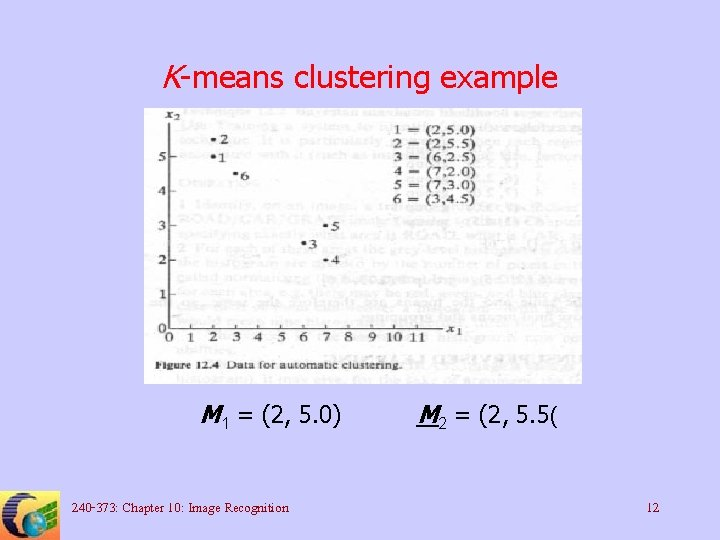K-means clustering example M 1 = (2, 5. 0) 240 -373: Chapter 10: Image Recognition M 2 = (2, 5. 5( 12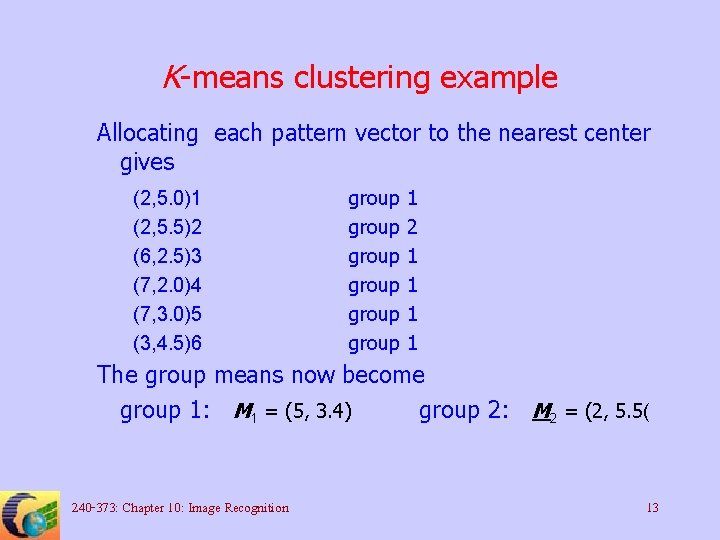K-means clustering example Allocating each pattern vector to the nearest center gives (2, 5. 0)1 (2, 5. 5)2 (6, 2. 5)3 (7, 2. 0)4 (7, 3. 0)5 (3, 4. 5)6 group group 1 2 1 1 The group means now become group 1: M 1 = (5, 3. 4) group 2: M 2 = (2, 5. 5( 240 -373: Chapter 10: Image Recognition 13K-means clustering example This gives new groupings as follows: (2, 5. 0)1 (2, 5. 5)2 (6, 2. 5)3 (7, 2. 0)4 (7, 3. 0)5 (3, 4. 5)6 group group 2 2 1 1 1 2 And the group means become group 1: M 1 = (6. 67, 2. 5) group 2: M 2 = (2. 33, 5. 0( Groupings now stay the same and the processing stops. 240 -373: Chapter 10: Image Recognition 14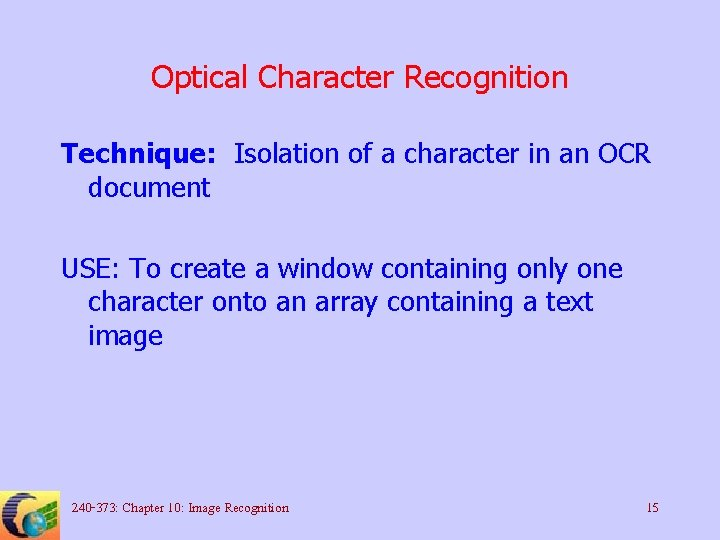Optical Character Recognition Technique: Isolation of a character in an OCR document USE: To create a window containing only one character onto an array containing a text image 240 -373: Chapter 10: Image Recognition 15Optical Character Recognition OPERATION: . 1 Assuming that the image is correctly oriented and the text is dark on a white background. 2 Calculate row sums of the pixel gray-level values. High row sums indicate a space between the rows. 3 Calculate column sums of the pixel gray-level values. High column sums indicate a space between the columns 240 -373: Chapter 10: Image Recognition 16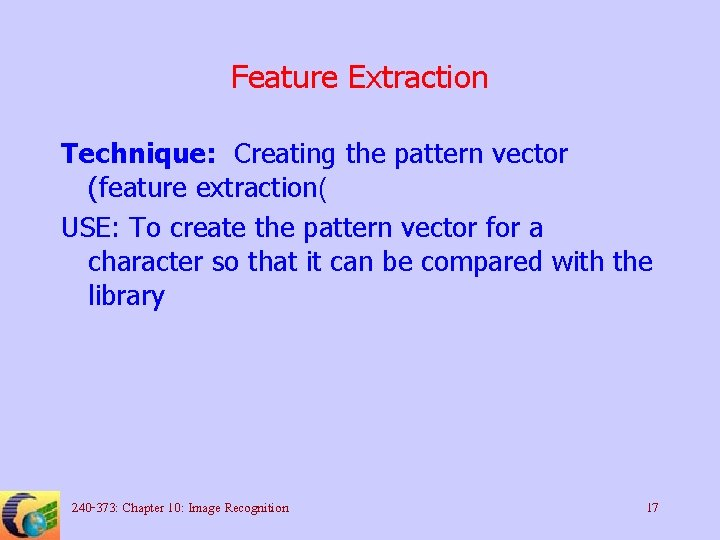Feature Extraction Technique: Creating the pattern vector (feature extraction( USE: To create the pattern vector for a character so that it can be compared with the library 240 -373: Chapter 10: Image Recognition 17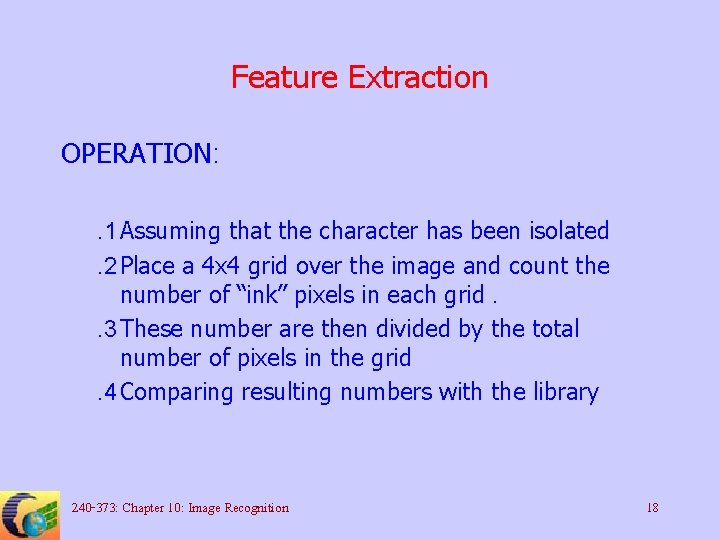Feature Extraction OPERATION: . 1 Assuming that the character has been isolated. 2 Place a 4 x 4 grid over the image and count the number of “ink” pixels in each grid. . 3 These number are then divided by the total number of pixels in the grid. 4 Comparing resulting numbers with the library 240 -373: Chapter 10: Image Recognition 18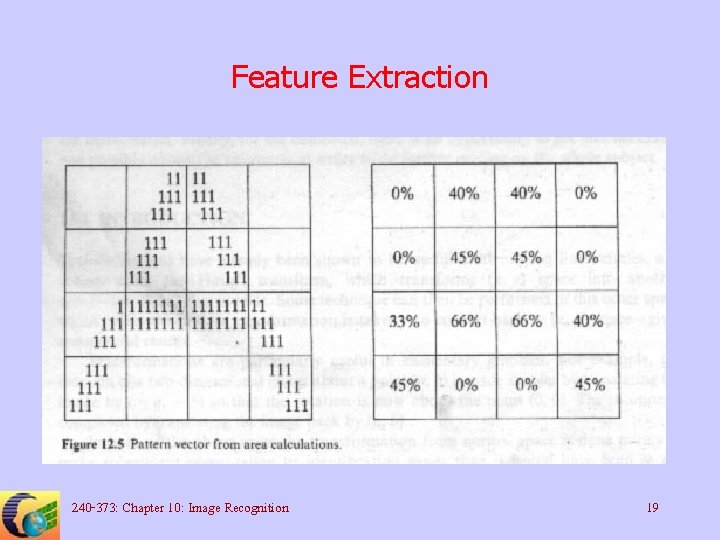Feature Extraction 240 -373: Chapter 10: Image Recognition 19